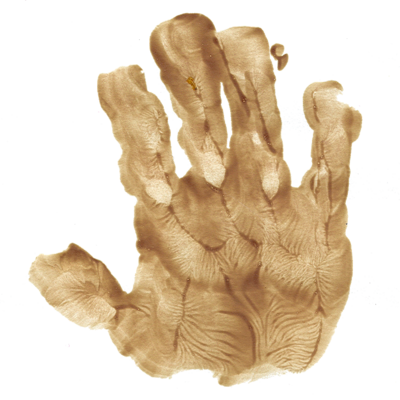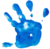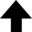Every spirit builds itself a house, and beyond its house a world … build therefore your own world.Ralph Waldo Emerson

The primary mechanism by which energetic (high velocity) charged particles lose energy as they pass through matter (solid, liquid, or gas) is through interactions with the electrons of that stopping medium. This is not surprising given the relative volumes occupied by electrons and nuclei. These interactions can be thought of as "collisions" between the incident charged projectile (modeled as a particle) and the target electrons. These collisions lead to ionization (i.e., the liberation of an electron from its "host" atom), excitation (i.e., the raising of an electron to a higher orbit or energy level around its atom), and/or bremsstrahlung, or the production of x-rays from a decelerating charged particle. When detecting particles with various instruments, we nearly always rely on the process of ionization in one form or another. Thus, it is important to understand how charged particles interact with matter, especially the matter out of which we build particle detectors. Electrons liberated (ionized) by the passage of a heavy charged particle are called $\delta$-rays (delta rays).

The loss of kinetic energy by a charged particle as it passes through matter can be described by an equation first derived classically by Niels Bohr, and then derived more rigorously using quantum mechanics by Hans Bethe and Felix Bloch. Today we refer to this equation as the Bethe-Bloch formula. The loss of kinetic energy per unit path length by the charged particle is called the stopping power. (In medical physics and radiation protection, it is referred to as the linear energy transfer, or LET). This loss of energy is primarily through collisions with the electrons of the medium. Because these electrons receive energy from the projectile, they are ionized, i.e., freed from their atomic positions. Ionization of electrons by the passage of a charged particle through the sensitive volume of a detector is the primary mode by which radiation is detected in most instruments.

The mean ionization potential $I$ is a quantity specific to the stopping medium. There are numerous published formulae for how to compute $I$ for a given material, but the resulting values are often less than satisfactory. The best practice is to use an experimentally determined value of $I$. (Please see Sternheimer Density Effect Parameters for a tool that will report $I$ in addition to a number of other relevant parameters for a given chemical formula.)

While the Bethe-Bloch formula can be used in principle to calculate the stopping power of any charged particle passing through matter, it must be modified in the case of electrons. There are several reasons for this:

• One must always consider special relativity when handling electron-electron interactions;
• An electron that acts as a projectile can lose nearly all of its kinetic energy in a single interaction with a target electron;
• Quantum mechanics tells us that we will not be able to distinguish between the projectile and the target electrons after the collision (they are identical particles);
• For a high energy electron that traverses a high $\bar{Z}$ stopping medium, radiative processes such as bremsstrahlung will be significant.

Some preliminary notes on the math: The two factors accounting for the Coulomb force and for the projectile can be challenging to work with. However, things become much easier once you realize that the classical electron radius $r_\text{e}$ is related to the factor referred to as "Coulomb" below: $(e^2 / 4 \pi \epsilon_0)^2 = (m_\text{e} c^2)^2 r_\text{e}^2$. Please see the appropriate section in the Range-Energy Calculator for more details on this and all the other symbols appearing in the Bethe-Bloch formula.

### References• "ESTAR: Stopping Power and Range Tables for Electrons." National Institute of Standards and Technology, Physical Measurement Laboratory.
• Bethe, H. and Heitler, W. (1934), "On the stopping of fast particles and on the creation of positive electrons," Proc. Royal Soc. Lond. 146, 83-112.

#### Acknowledgement

I wish to thank Dr. Eric Benton for the nuclear and particle physics notes that were used as inspiration for this page.

## Bethe-Bloch Formulae for Electrons

This program provides electron stopping power as a function of kinetic energy in a specified target material.
\displaystyle{ \begin{align*} \overbrace{ - \left \langle \frac{\mathrm{d} E}{\mathrm{d} x} \right \rangle_\text{c} }^\text{collision} = \overbrace{ \left( \frac{e^2}{4 \pi \epsilon_0} \right)^2 }^\text{Coulomb} \overbrace{ \frac{2 \pi}{m_\mathrm{e} c^2 \beta^2} }^\text{projectile} \overbrace{ \frac{\rho \bar{Z} N_\text{A}}{\bar{A}} }^\text{target} \left[ \ln{ \left( \frac{E (E + m_\mathrm{e} c^2)^2 \beta^2}{2 I^2 m_\mathrm{e} c^2} \right) } - \beta^2 + 1 - \left( 2 \sqrt{1 - \beta^2} + \beta^2 - 1 \right) \ln 2 \\ + \frac{1}{8} \left( 1 - \sqrt{1 - \beta^2} \right)^2 \right] \text{;} \end{align*} } $\displaystyle{ \underbrace{ - \left \langle \frac{\mathrm{d} E}{\mathrm{d} x} \right \rangle_\text{b} }_\text{bremsstrahlung} = \underbrace{ \left( \frac{e^2}{4 \pi \epsilon_0} \right)^2 }_\text{Coulomb} \underbrace{ \frac{\alpha (E + m_\mathrm{e} c^2)}{(m_\mathrm{e} c^2)^2} }_\text{projectile} \underbrace{ \frac{\rho \bar{Z}^2 N_\text{A}}{\bar{A}} }_\text{target} \left[ 4 \ln{ \left( \frac{2 (E + m_\mathrm{e} c^2)}{m_\mathrm{e} c^2} \right) } - \frac{4}{3} \right] \text{,} }$

where $\alpha$ is the fine-structure constant, and $\beta \equiv v /c = \sqrt{1 - \left[ m_\text{e} c^2 / (E + m_\text{e} c^2) \right]^2 }$ from special relativity.

Total stopping power = collision + bremsstrahlung, $\displaystyle{ \left \langle \frac{\mathrm{d} E}{\mathrm{d} x} \right \rangle = \left \langle \frac{\mathrm{d} E}{\mathrm{d} x} \right \rangle_\text{c} + \left \langle \frac{\mathrm{d} E}{\mathrm{d} x} \right \rangle_\text{b} }$.
Projectile

MeV0.01 ≤ $E_i$ ≤ 10 MeV

MeV10 ≤ $E_f$ ≤ 10000 MeV

MeV0.01 ≤ $\Delta E$ ≤ 1.0 MeV

Target:

0%
Current data point:

Legend: Collision (MeV‑cm2/g); Bremsstrahlung (MeV‑cm2/g); Total (MeV‑cm2/g)